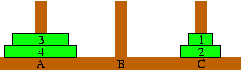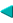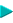The Towers of Hanoi and Adventures in Mathematics(page 100(2))The Towers of Hanoi SolutionDid you see the pattern that was developed in the previous page? Basically if we wanted to move a tower of size N (where N is a natural number greater than zero) from A to B, we: Move tower of size N minus 1 from A to C; Move the N-ring from A to B; Move tower of size N minus 1 from C to B. and if N is zero, we do nothing. So to move a tower of size N from A to B: If N is greater than zero then Move tower of size N minus 1 from A to C; Move the N-ring from A to B; Move tower of size N minus 1 from C to B. RecursionThe above instructions, or algorithm, is what we call recursive -- it calls itself. It is said to involve recursion. Those dictionary definitions weren't much help were they? Basically a recursive procedure, is one that invokes itself to solve a smaller version of the problem in order to solve the bigger problem. So in the Towers of Hanoi puzzle for a tower of size N, we use recursion to move towers of size N - 1, and as we recurse the N gets smaller until it reaches zero, which is trivial to solve. Neat isn't it? Recursion is a very important concept and is used a lot in computer programs.